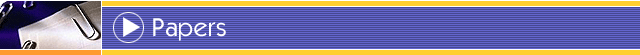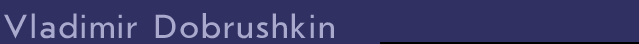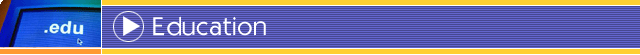Dr.Sc. Belarus Polytechnic Academy Minsk, Belarus 1992 Mechanical Engineering Ph.D. Belarus State University Minsk, Belarus 1978 Applied Mathematics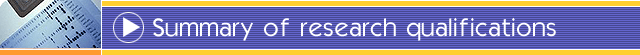Extensive experience in applied mathematics, solid, and fluid mechanics. Combination of strong theoretical background and mathematical simulation skills. Fields of activity include mathematical modeling of physical phenomena in solid mechanics, dynamic fracture mechanics, and two phase flow of incompressible fluid. Intensive applications of boundary element methods, finite element methods, and finite difference methods. Regularization and numerical calculation of singular and hypersingular integrals. Solid background in statistics. Good computer programming skills (Fortran, C/C++, PowerBuilder, and Matlab), UNIX experience (on SUN and SGI platform), and proficient in Windows applications (Word, Excel, Excess) and Software tools (HTML, Minitab, SAS, R, LaTeX, CASs).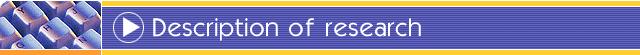Partial Differential Equations Wave equations with oblique boundary conditions: Scalar wave propagation problems with the Dirichlet or Neumann boundary conditions have been extensively studied. The mixed problem for the wave equation with an oblique derivative is ill-posed. The conditions of normal solvability were found. The exact solutions of initial value problems for wave equation have been constructed. Main publications: Dobrushkin, V.A. (1994). On solvability of mixed problem in a domain with an angle for wave equation. Sib. J. Diff. Equations, 3, No. 2, 3 - 10 (1994). Shock problems: The shock phenomena in solid mechanics is simulated as an initial-boundary value problem for PDE with discontinuous initial data. A rigorous solution of such problem was obtained and the uniqueness theorem was proved. Main publications: Dobrushkin, V.A., & Gaiduk, S.I. (1979). Solution of a problem in the theory of thermoelasticity related to mechanical and thermal shocks. English transl. in Differential equations, 15, 1165 - 1174; Dobrushkin, V.A. (1977). The uniqueness of the generalized solution of a certain problem in shock theory. English transl. in Differential Equations, 13 , 1061 - 1063. Resolvent method in abstract differential-operator equations and elastodynamics: A new method for solving linear boundary value problems for abstract operator -differential equations was developed, a variant of the boundary element method. The main advantage of the new method in comparison with this technique is the reduction of singularities. Main publication: Dobrushkin, V.A. (1983). A general boundary value problem for an abstract differential-operator equation, Dokl. Akad. Nauk BSSR, 27, 776 - 779 (in Russian; English summary). The dynamic problems of elasticity for domains with nondifferentiable boundaries pose special difficulties since the standard methods cannot be used reliably for the analysis of wave effects in these geometries. In these domains the effects of wave reflection and diffraction are strongly influenced by the irregular boundary. The solutions of several mixed problems in the dynamic theory of elasticity for the wedge-shaped domains with the coupled boundary conditions (when elastic potentials are not separated) were established. The necessary algorithms for the approximate calculation of these solution have been developed. Main publications: Dobrushkin, V.A. (1988). The boundary value problems in the dynamic theory of elasticity for the wedge-shaped domains. Minsk: Nauka I Technika (in Russian, monograph); Dobrushkin, V.A. (1984). The second boundary value problem of the plane theory of elasticity for a wedge. Dokl. Akad. Nauk SSSR, 1984, 279, No. 11 77 -79 (in Russian; English transl. in Soviet Phys. Dokl. 1984, 29, No. 11, 905 - 907)., 1061 - 1063. Numerical Methods Approximation of continual integrals: The appropriate numerical procedure to approximate the value of the continual integral with respect to several measures was developed. Main publication: Dobrushkin, V.A., & Likhoded, N.A. (1982). Approximate calculation of a continual integral with respect to a Cauchy measure with a weight in Hilbert space, 1982, No. 1, 104 - 106 (in Russian; English summary). Approximate evaluation of hypersingular integrals: A new method of regularization and approximate calculation of hypersingular integrals and integro-differential expressions with singularities was proposed. The estimates of quadrature errors were obtained. Main publications: Dobrushkin, V.A. (1992). Regularization in Marchaud form and approximate calculation of a class of integro- differential expressions. Izvestiya vuzov. Matematika, 1992, No. 9, .38 - 41 (in Russian; English transl. in Russian Mathematics. Izvest. VUZ, Matematika, 1992, No. 9, 34 - 37); Dobrushkin, V.A. (1993). Approximate calculation of a class of integro-differential expressions that describe the propagation of elastic waves. Prikladn. Mat. i Mech., No. 2, 164 -167. (in Russian; English transl. J. Appl. Math. Mechs. 1993, 57, 393 - 397). Mathematical Modelling The solution of initial-boundary value problem which simulates half-plane with a semi-infinite crack have been developed. Main publication: Clifton, R.J., & Dobrushkin, V.A. Elastic wave propagation in a half-space containing a buried crack. Submitted to Wave Motion. Simulation of the common motion of spherical bubbles in incompressible viscous unbounded fluid was developed. Main publications: Dobrushkin V.A., & Maxey M.R. . An improved boundary element formulation for the mothion of spherical bubbles. Submitted to J. Comp. Phys.. Dobrushkin, V.A., Maxey, M.R., & Likhoded, N.A. Fixed-size processor array for the implementation of the boundary element method in potential flow of spherical bubbles. Submitted to IEEE Transactions on Computers. Segregation of Mg Implanted into InAs: Influence of the Internal Elastic Stresses. Main publication: Dobrushkin, V.A., Velichko, O.I., Fedotov, A.K., Tsurko V.A. Diffusion and Defect Data, Part B: Solid State Phenomena, 2002, Vols.82-84, pp.569-574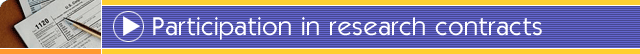1999 -- 2000 "Simulation of Coupled Diffusion of Impurity Atoms and Point Defects in the Vicinities of Interfaces Insulator-Semiconductor and Heterojunctions Semiconductor-Semiconductor" funded by the National Research Council's Collaboration in Basic Science and Engineering (COBASE). Objective: To formulate basic assumptions for the generalized models of impurity - defect system evolution in the vicinity of interfaces dielectric-semiconductor and heterojunctions semiconductor - semiconductor; and to develop an algorithm to solve a system of generalized impurity atoms and point defects diffusion equations. 1995 -- 1998 "Interaction of finite-sized particles and turbulence in dispersed two-phase flow" funded by the National Science Foundation (CTS-94-24169) Objective: To determine the potential flow of particles of arbitrary form and of ellipsoidal form. Description: An improved boundary element method is applied to simulate two-phase flow of particles of arbitrary form and of ellipsoidal form. 1994 -- 1996 "Dynamics of bubbly flows and the modification of turbulence by micro-bubbles" funded by the Office of Naval Research (ONR-N00014-91-J1340) Objective: To simulate multi-bubble motion with touching in viscous incompressible flow. Description: To solve Navier-Stokes equations an improved boundary element method was applied. It allows to reduce the problem under consideration to the Fredholm integral equation of the second kind without singularities. 1989 -- 1992 ``Computation of the stress-strain fields of rock under drill bits'', funded by the Petroleum University, Grozny, USSR (The leader of five-man team of developers). Objective: To determine the stress-strain field of rock under drill bits in order to accelerate the speed of drill in deep bore hole. Description: The process of drilling was simulated by the solution of the system of partial differential equations of elastodynamics and Newtonian flow to describe the problem of crack propagation under penetrating viscous liquid. 1987 -- 1989 ``Analysis and calculations of thermal processes in optical non-linear semiconductor structures'' funded by the Ministry of Defense, Minsk, USSR (The leader of four-man team of developers). Objective: To analyze the temperature and translucency effects of a laser beam penetrating a multi-layer semiconductor material. Description: The temperature distribution in a multi-layer semiconductor material was numerically analyzed by using finite difference schemes. This knowledge was applied to figure out the hysteresis of the phenomena. Experimental results agreed well with theoretical calculations. 1985 -- 1987 ``Calculation of the stress-strain fields of metal-ceramic solids in thermal flux'' funded by the Soviet Air Force for Space Shuttle ``Buran''. Leningrad, USSR (The leader of four-man team of developers). Objective: To determine the high temperature expansion properties of metal-ceramic solids. Description: The focus of the project was to allow the ``Buran'' Shuttle to deflect reentry atmosphere friction heat. The Lame method and the Finite Element Method were used to determine the stress-strain fields in a solid consisted of metal and ceramic. The numerical results matched the experimental ones. 1978 -- 1980 ``Numerical analysis of branching pipeline oscillations of for nuclear power stations'' funded by the Polzunov Power Research Institute, Leningrad, USSR (The leader of four-man team of developers). Objective: To determine the locations of supporters for branching pipelines in order to prevent their damage due to earthquake Description: The dynamic behavior of oscillated pipelines were modeled as a system of ordinary differential equations and numerical simulated with feedback constrains algorithm. This algorithm used the calculated eigenvalues to analyze and determine the necessary positions of pipeline supporters.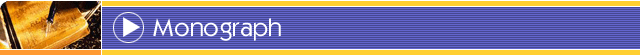Dobrushkin V.A. (2022). Applied Differential Equations. The Primary Course, Chapman & Hall/CRC, 2022, second edition, ISBN-13: 978-1439851043  Zwillinger, D. and Dobrushkin V.A. (2021). Handbook of Differential Equations, Fourth Edition, Chapman & Hall/CRC, 2021, fourth edition, ISBN-13: 978-0-367-25257-1  Dobrushkin V.A. (2019). Mathematics of Social Choice and Finance, Kendall Hunt Publishing, 2019, second edition, ISBN-13: 978-1-5249-8959-0  Dobrushkin V.A. (2017). Applied Differential Equations with Boundary Value Problems, Chapman & Hall/CRC, 2017, ISBN-13: ‎ 1498733654  Dobrushkin V.A. (2014). Applied Differential Equations. The Primary Course, Chapman & Hall/CRC, 2014, ISBN-10:1439851042, ISBN-13: 978-1439851043  Dobrushkin V.A. (2014). Mathematics of Social Choice and Finance, Kendall Hunt Publishing, 2014, ISBN-10: 1465250999, ISBN-13: 978-1465250995  Dobrushkin V.A. (2009). Methods in Algorithmic Analysis (CRC Computer and Information Science Series), Chapman & Hall/CRC, 2009, ISBN-10: 1420068296, ISBN-13: 978-1420068290   Dobrushkin V.A. (1988). The boundary value problems in the dynamic theory of elasticity for the wedge-shaped domains. Minsk: Nauka i Technika (in Russian).